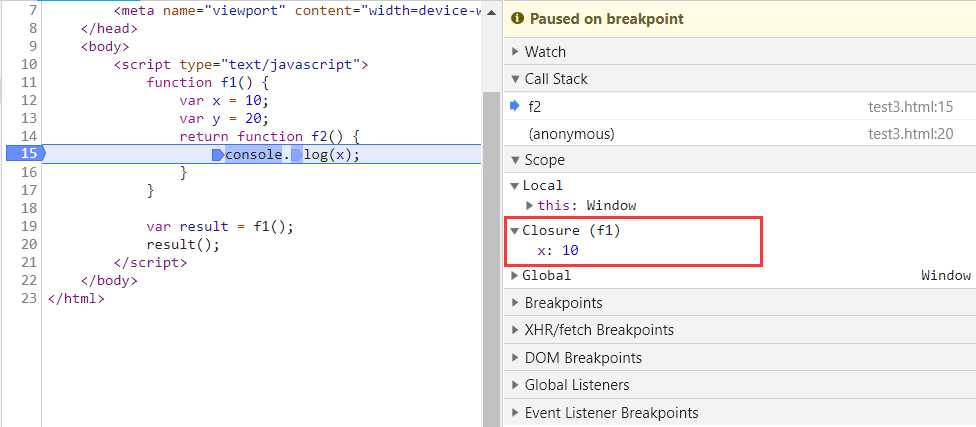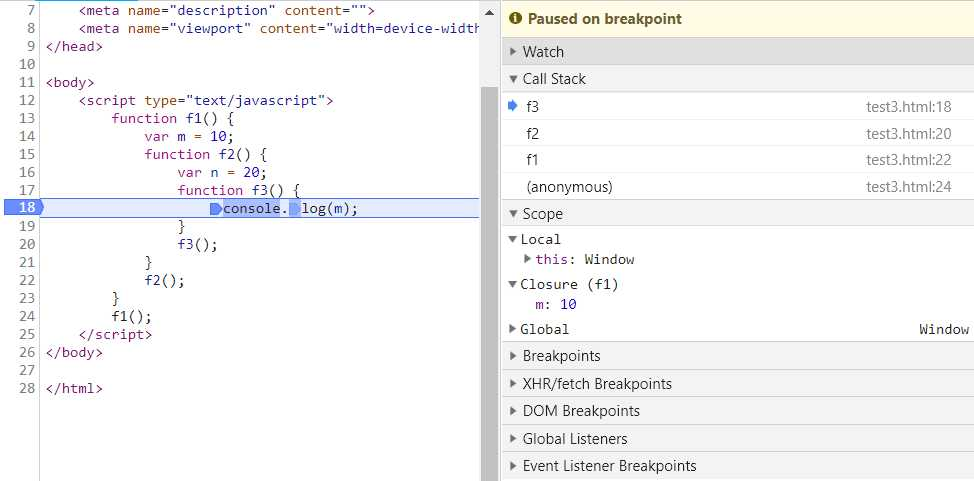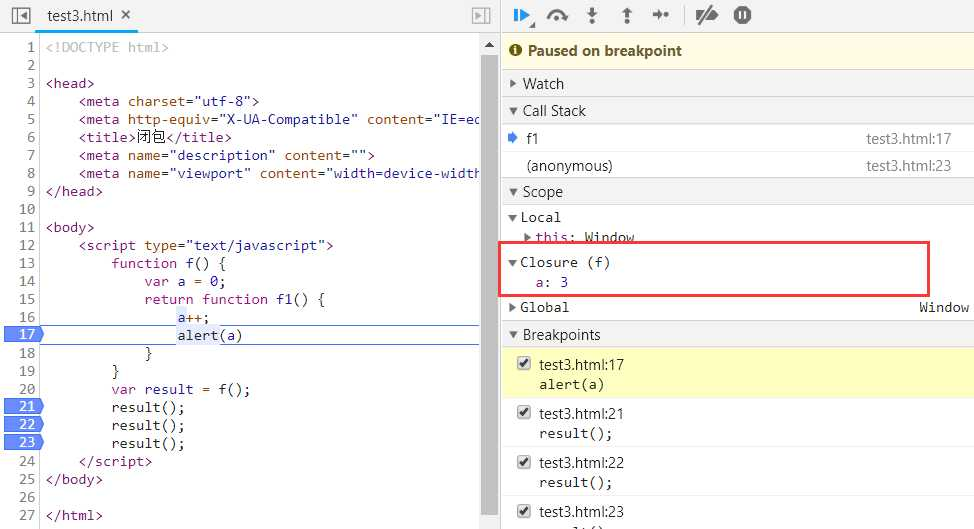# 1.什么是闭包？

``` 1 <body>
2     <script type="text/javascript">
3         function f1() {
4             var x = 10;
5             var y = 20;
6             return function f2() {
7                 console.log(x);
8             }
9         }
10
11         var result = f1();
12         result();
13     </script>
14 </body>```# 2.闭包是什么时候创建的？

``` 1 <body>
2     <script type="text/javascript">
3         function f1() {
4             var m = 10;
5             function f2() {
6                 var n = 20;
7                 function f3() {
8                     console.log(m);
9                 }
10                 f3();
11             }
12             f2();
13         }
14         f1();
15     </script>
16 </body>```# 3.闭包的好处

## 3.1减少全局变量

``` 1 <body>
2     <script type="text/javascript">
3         var a = 0;
4         function f() {
5             a++;
7         }
8         f();
9         f();
10         f();
11     </script>
12 </body>```

``` 1 <body>
2     <script type="text/javascript">
3         function f() {
4             var a = 0;
5             return function f1() {
6                 a++;
8             }
9         }
10         var result = f(); //f()的返回值是一个函数f1()
11         result(); //所以这里调用result()就等于调用绑定了变量a的f1()
12         result();
13         result();
14     </script>
15 </body>```## 3.2减少传递给函数的参数数量

``` 1 <body>
2     <script type="text/javascript">
3         //创建一个工厂方法，接收一个base参数，返回一个基于这个参数的计算方法
4         function callFactory(base) {
5             //计算方法，接收一个参数，用于计算范围
6             return function(max) {
7                 var total = 0;
8                 for (let i = 0; i < max; i++) {
9                     total += i;
10                 }
11                 //计算方法的返回值
13             }
14         }
15         var adder = callFactory(2);//这里调用callFactory(2)工厂方法返回了一个基于参数2的function(max)
18     </script>
19 </body>```

## 3.3封装

``` 1 <body>
2     <script type="text/javascript">
3         (function() {
4             var m = 0;
5             function getM() {
6                 return m;
7             }
8             function setM(param) {
9                 m = param;
10             }
11             window.g = getM; //由于在浏览器中执行，这里先挂载在window对象上
12             window.s = setM;
13         })();
14         s(12);
16     </script>
17 </body>```

# 4.使用闭包的注意点

1. 捕获的变量只是个引用，而不是复制。
2. 父函数每调用一次，都会产生一个新的闭包，因为函数每次调用都会创建新的词法环境。
3. 使用闭包解决循环中的回调函数的异步问题。

``` 1 <body>
2     <div id="1">1</div>
3     <div id="2">2</div>
4     <div id="3">3</div>
5     <script type="text/javascript">
6         for (var i = 1; i <= 3; i++) {
7             var di = document.getElementById(i);
8             di.onclick = function () {
10             }
11         }
12     </script>
13 </body>```

``` 1 <body>
2     <div id="1">1</div>
3     <div id="2">2</div>
4     <div id="3">3</div>
5     <script type="text/javascript">
6         for (var i = 1; i <= 3; i++) {
7             var di = document.getElementById(i);
8             di.onclick = (function (id) { //每次循环时，通过一个立即调用的函数，将i与函数绑定形成不同的闭包
9                 return function() {
11                 }
12             })(i);
13         }
14     </script>
15 </body>```

JS闭包

(0)
(0)

© 2014 bubuko.com 版权所有 鲁ICP备09046678号-4